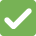# Calculations

We love them!

When using the API you might notice that the data you send to us differs with a 1-10 cent difference. This is because we recalculate your data to make sure its correct.

# Why does Billit do this?

Billit calculates based on the taxable amount. This is a standard coming from the EU.
In Belgium you are allowed to use Both ways

• Order line based
• Taxable amount based.

Since the EU way is more specific and correct we use that one. This also makes it easy for Non Belgian customers to use our API.

# What can you do to have the required result?

When creating orderliness you can chose which value you want to be leading the calculations.

VatIncl or VatExcl this value can be set on order line level allowing you to chose which value is master.

# What is different from Billit its calculations and others

There are 2 ways of calculating invoice lines.
See the table below as orderlines. We will use the same for both calculations.

Order line TitlePriceVat
Phone10021%
Food506%
Drinks7512%
Computer60021%

Order line based ❌

The order line based method will go line for line and calculate the vat percentage and sum them up.

``````100 + (100*0.21) = 121
+
50 + (50*0.06) = 53
+
75 + (75*0.12) = 84
+
600 + (600*0.21) = 726
=
984
``````

As you can see we calculate the invoice line and add it to the sum.

Vat based (taxable amount) Billit Uses this oneThe Taxable amount method will combine all percentages and then sum the results together,

``````(100+600) + ((100+600)*0.21) = 847
+
50 + (50*0.06) = 53
+
75 + (75*0.12) = 84
=
984
``````

In the case above you can see that here is no difference, since there are no cents used. This is mainly to show you how the 2 methods work.